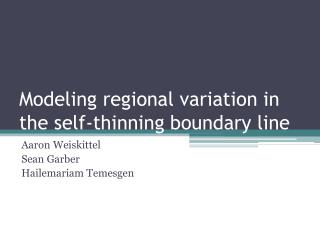DownloadDownload PresentationModeling regional variation in the self-thinning boundary line

# Modeling regional variation in the self-thinning boundary line

Télécharger la présentation## Modeling regional variation in the self-thinning boundary line

- - - - - - - - - - - - - - - - - - - - - - - - - - - E N D - - - - - - - - - - - - - - - - - - - - - - - - - - -
##### Presentation Transcript

1. Modeling regional variation in the self-thinning boundary line Aaron Weiskittel Sean Garber Hailemariam Temesgen

2. Introduction • Although self-thinning constraints may not be needed for individual tree growth models (Monserud et al. 2005; For. Sci. 50: 848), they are still important for: • Stand-level projections • Developing stand management diagrams • Understanding basic stand dynamics

3. Introduction • Size-density relations have been quantified for a variety of species and it has been suggested that: • A universal slope exists (-3/2) • Intercept varies by species, but is not influenced by other factors • Previous analyses have relied on ordinary least squares (OLS) or principal components analysis (PCA) to examine trends • Assumptions are violated and tests of parameter significance are invalid

4. Introduction • Zhang et al. (2005; CJFR 35: 1507) compared several different methods for estimating the self-thinning boundary line • OLS and PCA performed the poorest • sensitive to the data subjectively selected for fitting • may produce lines with the inappropriate slope • Statistical inference is difficult with quantile regression and deterministic frontier functions • Stochastic frontier functions (SFF) performed the best

5. Introduction • Bi (2001; For. Sci. 47, 361) used SFF to examine the self-thinning surface in Pinusradiata • SFF successfully separated the effects of density-dependent and density-independent mortality • SFF allows statistical inferences on the model coefficients • Generalized model form proposed: • B = β0Sβ1Nβ2 • where B is stand biomass per unit area, N is stand density, S is relative site index, and βi’sareparameters

6. Objectives • Utilize SFF to examine maximum size-density relations in coastal Douglas-fir, red alder, and lodgepole pine • Test the generality of Bi’s (2001) model • Examine the influence of other covariates • Compare the results to a more traditional approach

7. Analysis • Used Frontier v4.1 (Coelli 1996) and R library micEcon to fit the SFF • ln(TPA) = β10 - β11ln(QMD) + ε11 • QMD is quadratic mean diameter and TPA is trees per acre • Compared to fits obtained using quantile regression • Maximum stand density index (SDImax) was estimated for each plot and regressed on other covariates similar to Hann et al. (2003) • Significance of covariates evaluated using log-likelihood ratio tests

8. Data

9. Stochastic frontier analysis • Used in econometrics to study firm efficiency and cost & profit frontiers • Model error has two components • Random symmetrical statistical noise • Systematic deviations from the frontier • Qit = exp(ß0 + ß1 ln(xit)) * exp(vit) * exp(-uit) Deterministic component Inefficiency Random noise

10. Stochastic frontier analysis • Fit using maximum likelihood • u and v are assumed to be distributed independently of each other and the regressors • u represents the difference in stand density at any given point and the estimated maximum density • Eliminates the subjectively of choosing stands that other techniques rely on

11. Results: Maximum stand density • Plot-specific SDImax showed no relationship with any other covariates

12. Results: Self-thinning boundary line • Stochastic frontier analysis and quantile regression produce significantly different results

13. Results: Self-thinning boundary line • Likelihood ratio tests indicated that the inclusion of site index improved the model for Douglas-fir and red alder, but not for lodgepole pine • The effect of fertilization in Douglas-fir was insignificant • Red alder was also influenced by slope and aspect as well as soil water holding capacity

14. Conclusion • Stochastic frontier functions proved very useful for this type of analysis and provided insights that other statistical techniques obscure • SDImaxvalues higher in this analysis slightly different than previously published values • Lower for Douglas-fir, but higher for red alder and lodgepole pine • Douglas-fir and red alder support Bi’s general model, but lodgepole does not • Site index only capture some of the variation for red alder

15. Next Steps • Compare plantation to natural stands • Use a more extensive red alder database • Western Hemlock

16. Acknowledgements • Thanks to SMC, SNCC, HSC, BC Ministry of Forests and their supporting members for access to the data